Courses

# MCQ (Previous Year Questions) - Circle (Competition Level 1)

## 24 Questions MCQ Test Mathematics Past Year Papers | MCQ (Previous Year Questions) - Circle (Competition Level 1)

Description
This mock test of MCQ (Previous Year Questions) - Circle (Competition Level 1) for JEE helps you for every JEE entrance exam. This contains 24 Multiple Choice Questions for JEE MCQ (Previous Year Questions) - Circle (Competition Level 1) (mcq) to study with solutions a complete question bank. The solved questions answers in this MCQ (Previous Year Questions) - Circle (Competition Level 1) quiz give you a good mix of easy questions and tough questions. JEE students definitely take this MCQ (Previous Year Questions) - Circle (Competition Level 1) exercise for a better result in the exam. You can find other MCQ (Previous Year Questions) - Circle (Competition Level 1) extra questions, long questions & short questions for JEE on EduRev as well by searching above.
QUESTION: 1

### The square of the length of tangent from  (3, –4) on the circle x2 + y2 – 4x – 6y + 3 = 0 [AIEEE-2002]

Solution:

S1 = 9 + 16 – 12 + 24 + 3 = 40

QUESTION: 2

### If the two circles (x – 1)2 + (y – 3)2 = r2 and  x2 + y2 – 8x + 2y + 8 = 0 intersect in two distinct points, then [AIEEE-2003]

Solution: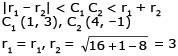5 < r + 3
r > 2
|r1 - r2I < C1 C2
|r- 3| < 5
-5 < r - 3 < 5
-2 < r < 8
So finally 2 < r < 8

QUESTION: 3

### The lines 2x – 3y = 5 and 3x – 4y = 7 are diameters of a circle having area as 154 sq. units. Then the equation of the circle is [AIEEE-2003]

Solution:

πr2 = 154, 2x - 3y = 5 x 3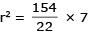On solving 2x - 3y = 5 & 3x - 4y = 7 we get, x = 1, y = -1
So centre (1, -1)
equation of circle (x - 1)2 + (y + 1)2 = 72
x2+ y2 - 2x + 2y = 47

QUESTION: 4

If a circle passes through the point (a, b) and cuts the circle x2 + y2 = 4 orthogonally, then the locus of its centre is

-[AIEEE-2004]

Solution:

let (h, k) be centre of circle equation of circle
(x - h)2 + (y - k)2 = (a - h)2 + (b - k)2
x2 - 2xh + h2 + y2 - 2yk + k2
= a2 + h2 - 2ah + b2 + k2 - 2bk
x2 + y2 - 2xh - 2yk + 2ah + 2bk - a2 - b2 = 0
For orthogonal
2g1g2 + 2 ff2 = c1 + c2
2h. 0 2k. 0
= 2ah + 2bk - a2 - b2 - p2 = 0
2ah + 2bk - (a2 + b2 + p2) = 0
locus of (h, k) is tax + 2by - (a2 + b2 + p2) = 0

QUESTION: 5

A variable circle passes through the fixed point A(p, q) and touches x-axis. The locus of the other end of the diameter through A is -

[AIEEE-2004]

Solution:

Let the equation of the circle is (x - h)2 + (y - k)2 = k2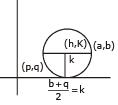(2k)2 = (a - b)2 + (b - 2)2
(b + q)2 = (a - p)2 + b2 + q2 - 2bq
(a - p)2 = 4bq
(x - p)2 = 4pq

QUESTION: 6

If the lines 2x + 3y + 1 = 0 and 3x – y – 4 = 0 lie along diameters of a circle of circumference 10π, then the equation of the circle is -

[AIEEE-2004]

Solution:

2x + 3y + 1= 0
3x - y - 4 = 0 x 3
11x - 11= 0
x = 1
y = -1
2πr = 10π
r = 5
(x - 1)2 + (y + 1)2 = 52
so x2 + y2 - 2x + 2y - 23 = 0

QUESTION: 7

If the circles x2 + y2 + 2ax + cy + a = 0 and x2 + y2 – 3ax + dy – 1 = 0 intersect in two distinct point P and Q then the lines 5x + by – a = 0 passes through P and Q for -

[AIEEE-2005]

Solution:

PQ is common chord equation of PQ is S1 - S2 = 0
⇒ 5ax + (c - d) y + a + 1 = 0 also PQ 5x + by - a = 0 both are identical so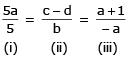By (i) & (iii)
-a2 = a + 1
a2 + a + 1 = 0
which is always > 0
So option B is correct

QUESTION: 8

A circle touches the x-axis and also touches the circle with centre at (0, 3) and radius 2. The locus of the centre of the circle is -

[AIEEE-2005]

Solution:

Let center be (h, k)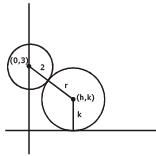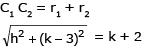h2 + k2 - 6k + 9 = k2 + 4k + 4
h2 = 10k - 5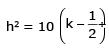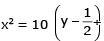which is a parabola

QUESTION: 9

If a circle passes through the point (a, b) and cuts the circle x2 + y2 = p2 orthogonally, then the equation of the locus of its centre is -

[AIEEE-2005]

Solution:

let (h, k) be centre of circle equation of circle
(x - h)2 + (y - k)2 = (a - h)2 + (b - k)2
x2 - 2xh + h2 + y2 - 2yk + k2
= a2 + h2 - 2ah + b2 + k2 - 2bk
x2 + y2 - 2xh - 2yk + 2ah + 2bk - a2 - b2 = 0
For orthogonal
2 g1g2 + 2 f1 f2 = c1 + c2 2h. 0 + 2k. 0
= 2ah + 2bk - a2 - b2 - p2 = 0
2ah + 2bk - (a2 + b2 + p2) = 0 locus of (h, k) is tax + 2by -(a2 + b2 + p2) = 0

QUESTION: 10

If the pair of line ax2 + 2(a + b)xy + by2 = 0 lie along diameters of a circle and divide the circle into four sectors such that the area of one of the sectors is thrice the area of another sector then

[AIEEE-2005]

Solution:

angle between pair of straight line is given by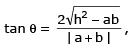ax2 + 2(a+b) xy + by2 = 0
h = (a+b)
4θ = π ⇒ θ = π/4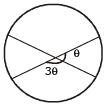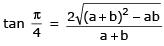(a+b)2 = 4(a+b)2 - 4ab
3(a+b)2 - 4ab = 0
3a2 + 3b2 + 2ab = 0

QUESTION: 11

If thel ines 3x – 4y – 7 = 0 and 2x – 3y – 5 = 0 are two diameters of a circle of area 49 π square units, the equation of the circle is -

[AIEEE-2006]

Solution:
QUESTION: 12

The triangle PQR is inscribed in the circle, x2+y= 25. If Q and R have co-ordinates (3, 4) & (–4, 3) respectively, then √QPR is equal to

[JEE 2000(Scr.), 1 + 1]

Solution:

x2 + y2 = 25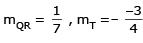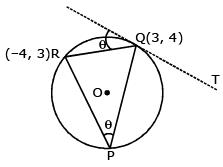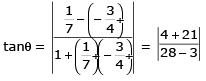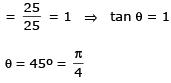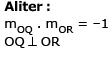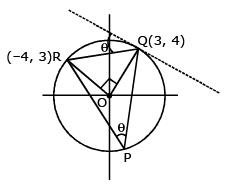∴ angle substended by a chord at centre is double of the angle substanded at point on the opposite side of cirlce.
∠QPR = 4

QUESTION: 13

If the circles, x2 + y2 + 2x + 2ky + 6 = 0 & x2 + y2 + 2ky + k = 0 intersect orthogonally, then ‘k’ is

Solution:

x2+y2+2x+2ky+6 = 0
x2+y2+2ky+k = 0
cuts orthogonally
⇒ 2.1.0 + 2.k.k = 6 + k
⇒ 2k2 - k - 6 = 0
(k - 2) (2k + 3) = 0 k = 2,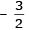QUESTION: 14

Let PQ and RS be tangents at the extremities of the diameter PR of a circle of radius r. If PS and RQ intersect at a point X on the circumference of the circle then 2r equals.

[JEE 2001 (Scr.), 1]

Solution:

In ΔPQR
PQ/2r = tan θ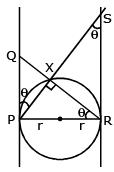& In ΔPRR
PR/RS = tan θ
⇒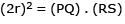⇒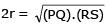QUESTION: 15

Let 2x2 + y2 – 3xy = 0 be the equation of a pair of tangents drawn from the origin 'O' to a circle of radius 3 with centre in the first quadrant. If A is one of the points of contact, find the length of OA.

Solution:

Pair of lines
2x2 - 3xy + y2 = 0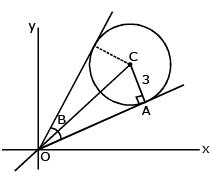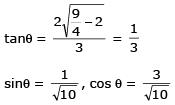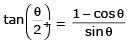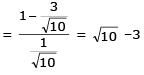In ΔOAC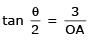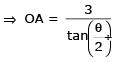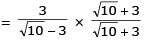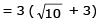QUESTION: 16

If the tangent at the point P on the circle x2 + y2 + 6x +6y = 2 meets the straight line 5x – 2y + 6 = 0 at a point Q on the y-axis, then the length of PQ is

Solution:

x2 + y2 + 6x + 6y - 2 = 0
Q ≡ (0,3)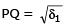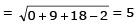QUESTION: 17

If a > 2b > 0 then the positive value of m for which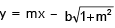i s a common tangent to  x2 + y2 = b2 and (x - a)2 + y2 = b2 is

[JEE 2002 (Scr.)]

Solution:

x > 2b > 0
x2 + y2 = b2, (x - a)2 + y2 = b2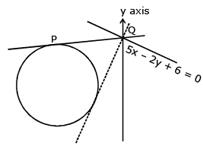common tangent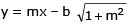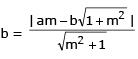⇒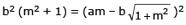⇒ b2 (m2 + 1) = a2m2 + b2 (1 + m2) - 2abm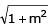m ≠ 0 or (ma)2 = (2b)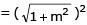∴ m > 0 m2 (a2 - 4b2) = 4b2
⇒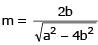QUESTION: 18

The radius of the circle, having centre at (2, 1), whose one of the chord is a diameter of the circle x2 + y2 – 2x – 6y + 6  = 0

[JEE 2004(Scr.)]

Solution:

S1 ≡ x2 + y2 - 2x - 6y + 6 = 0
center r1 (1, 3), r2 = 2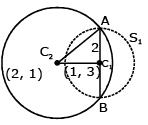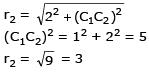QUESTION: 19

Tangents drawn from the point P(1, 8) to the circle x2 + y2 – 6x – 4y – 11 = 0 touch the circle at the points A and B. The equation of the circumcircle of the triangle PAB is

Solution:

x2 + y2 - 6x - 4y - 11 = 0
PACD is a cyclic quadrialteral C(3, 2)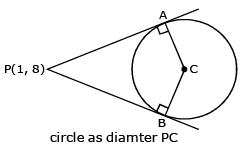(x - 1) (x - 3) + (y -8) (y - 2) = 0
x2 - 4x + 3 + y2 - 10y + 16 = 0
⇒ x2 + y2 - 4x - 10y + 19 = 0

QUESTION: 20

The centres of two circles C1 and C2 each of unit radius are at a distance of 6 units from each other. Let P be the mid point of the line segment joining the centres of C1 and C2 and C be a circle touching circles C1 and Cexternally. If a common tangent to C1 and C passing through P is also a common tangent to C2 and C, then the radius of the circle C is

[JEE 2009]

Solution:

(PM)2 = 32 - 12 = 8
PQ2 = (r + 1)2 - 32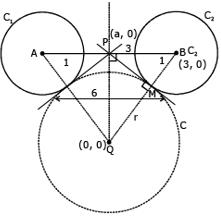IN ΔPMQ
PQ2 = (PM)2 + r2
= 8 + r2
r2 + 2r + 1 - 9 = 8 + r2
2r = 16
r = 8

QUESTION: 21

Two parallel chords of a circle of radius 2 are at a distance 3 + 1 apart. If the chords subtend at the center, angles of π/k andk 2π/k , where k > 0, then the value of [k] is
{Note : [k] denotes the largest integer less than or equal to k}

[JEE 2010]

Solution: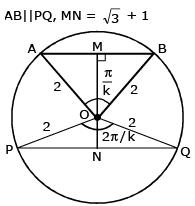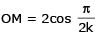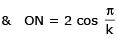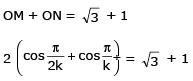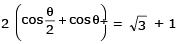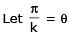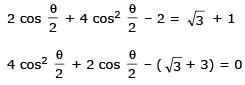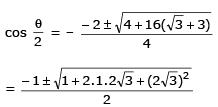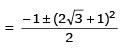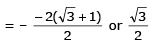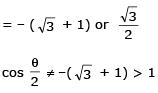∴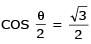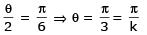⇒ k = 3

QUESTION: 22

The circle passing through the point (–1, 0) and touching the y-axis at (0, 2) also passes through the point

Solution:

Equation of cirde using family of circle (x - a)2 + (y - 2)2 + λ x = 0
put (-1, 0) to get λ & check from options

QUESTION: 23

The locus of the mid-point of the chord of contact of tangents drawn from points lying on the straight line 4x – 5y = 20 to the circle x2 + y2 = 9 is

[JEE 2012]

Solution: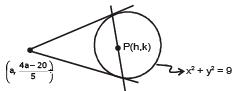Equation of chord of contact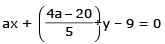5ax + 4ay - 20y - 45 = 0
5ax + (4a - 20) y - 45 = 0
equation of chord of mid point hx + ky = h2 + k2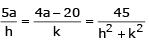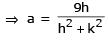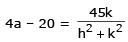put the value of a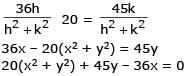QUESTION: 24

A tangent PT is drawn to the circle x2 + y2 = 4 at the point P (√3, 1) . A straight line L, perpendicular toPT is a tangent to the circle (x – 3)2 + y2 = 1. A possible equation of L is

[JEE 2012]

Solution: